GFG App
Open AppBrowser
Continue

A force is a push or pull upon a body of any mass resulting from the body’s interaction with another body. Whenever there is an interaction between two body, there is a force upon each of the bodies. Forces only exist as a result of an interaction. When an object is either pushed or pulled is known as a force. A force due to an action-generated motion in an object.

Force also has direction; which means that it is a vector quantity if the magnitude or direction changes, it directly affects the force. If the direction of force and the direction of moving object is opposite then it decreases the force. If an object is in motion, then an external force may change, the state or the direction of motion of that object. The state of motion in an object is usually explained by its speed and direction of motions.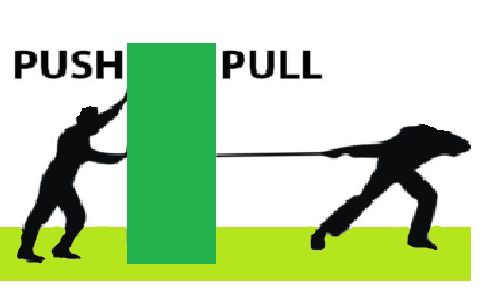### What is Force?

Force can be defined as a push or a pull on an object of any mass and on any surface. For any force applied there is a change in motion, state, shape, size, etc. of an object. The force has both magnitude and direction, as it is a vector quantity. It can be measured using a spring balance by placing the object at its hook end.

Forces are either long-range or short-range order. Electrostatic forces are long-range forces as well as the gravitational force is also long-range force.

The state of ‘rest’ of an object is considered to be when object or body has zero speed, as −

1. An object cannot move by itself.

2. An object cannot change its speed by itself.

3. An object cannot change its direction by itself.

4. An object cannot change by itself.

5. A force may make an object move from rest.

6. A force may change the speed of a moving object.

7. A force may change the direction of a moving object.

8. A force may change the shape of an object.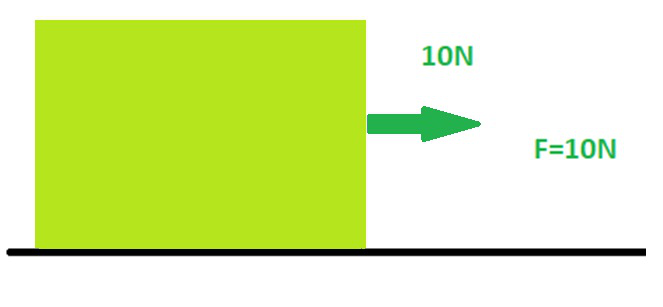## Exploring Forces

A force can change the motion of an object, i.e. a force can make a stationary body move; it can make a moving object stop; it can increase or decrease the speed of the moving body.

### Forces in the same direction

The Forces which are applied to an object in the same direction always get added to one another. If two forces are acting on an object in the same direction then the net force is equal to the sum of the two forces on it. In this case, it always results in a stronger force than either of the individual forces alone.

Example: Two people pushing a heavy car or any heavy box in the same direction.

When a heavy good is transported from one place to another many people apply forces in the same direction to move the box.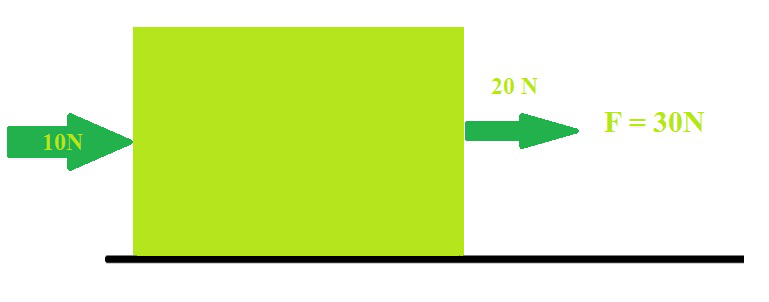### Forces in the opposite direction

If the two forces are acting in opposite directions on an object, the net force acting on it is always the difference between the two forces and acting in the direction of the force that has high magnitude.

Examples: Two people pushing a heavy load in the opposite direction. The net force on an object is zero if the two forces acting on it in opposite directions are equal in magnitude and if any unequal forces are applied then the box moves in a direction in which greater force was applied.

People playing in the tug of war pull the rope on opposite sides and whichever team pulls it with a greater force wins.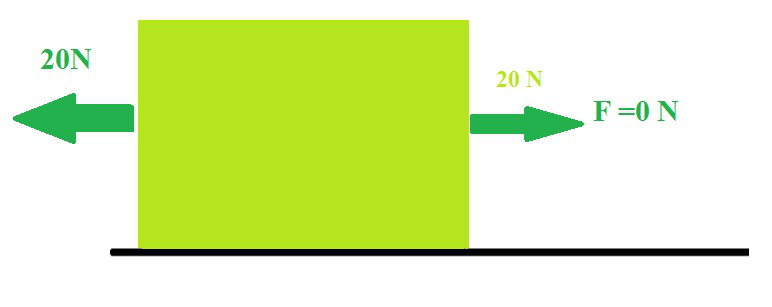### Magnitude and Direction of a Force

A force could be higher or smaller than another depending upon the strength of magnitude. To completely define a force, we have to also specify the direction in which it acts on the body. If the direction or the magnitude of the applied force changes, its effect also changes accordingly.

### Effect of Force

A Force can Change the State of Motion

A change in either the speed of an object, or in the direction of motion, or both, is described as a change in its state of motion.

Example: A fielder catches a cricket ball coming to him with great speed and brings it to rest. A batsman hits the ball and changes its direction of motion.

A Force can Change the Shape of an Object

The shape of an object can be changed using force. We use it to obtain the required shape of the object in some cases.

Example: Upon Pressing an inflated balloon by two hands compresses the balloon. While making the dough we change the shape of the dough as required.

## Types of Forces

Basically, forces are of two types:

1. Contact forces
2. Non-contact forces or At a distance forces

### Contact Force

A contact force is any force that requires contact with the surface. Contact forces are ubiquitous and are responsible for almost every visible interaction between macroscopic matters. Some of the everyday examples where contact forces are at work are pushing a car up a hill or kicking a ball across a room.

Basically, there are two types of contact forces;

1. Frictional force
2. Muscular force

### Frictional force

Frictional force is known as an opposing force that opposes the motion of one body over the surface of another body. It is absolutely necessary for the motion of any object over another. It is like a necessary evil that harms and helps. Its dual function can be understood by the example of walking, we are able to walk because there is friction, and we are walking at a certain low speed because of the opposing force provided by friction.

Friction is a force between two surfaces that are sliding, in contact, or very much trying to slide, across each other. Friction always slows a moving object down. The rougher the surface gets, the more friction produced. Friction also produces heat.

The force of friction has the following formula:Where F= force of frictioncoefficient of friction

N= normal reaction force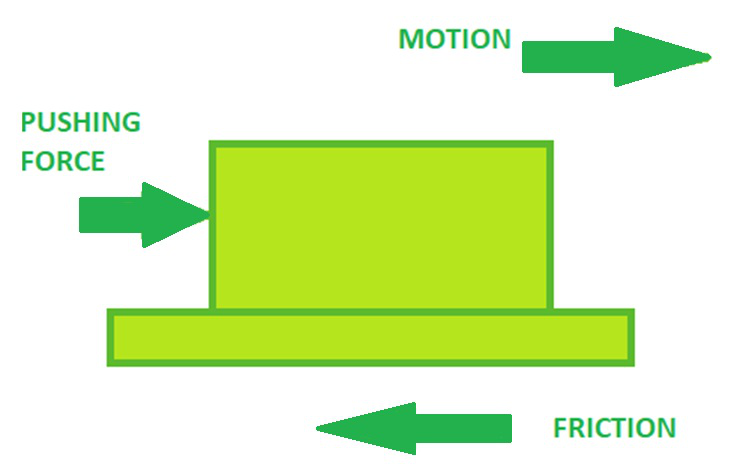### Muscular force

Force that is exerted by muscles is called the Muscular force of a body. All our activities of the human body like walking, lifting, running, bending are because of muscular force. It is a contact force because muscular force can only be exerted on physical contact like walking, running, jumping, and so on or Muscular force is called contact force because muscles are in direct contact with the object or body on which force is applied while applying force. In simple words, it is a force that results because of the action of muscles and is a contact force since there is contact between the surfaces. Muscular force required whenever any movement of the body occurs. Getting up from a seat, Making food, Running, Strolling, lifting, getting up from a seat, crossing a leg, and so on all require muscular force.

### Non-Contact Forces

A non-contact force is a force that acts on an object without making contact with it. One of the familiar non-contact force is gravity, which confers weight. In contrast, a contact force is a force applied to a body by another body that is in contact with it or in other words, Forces that arise without the contact of 2 or more objects involved. The types of non-contact forces are:

1. Magnetic Force
2. Electrostatic Force
3. Gravitational force.

### Magnetic force

Magnetic force refers to attraction or repulsion that arises between the electrically charged particles because of their motion. It refers to the force responsible for the action of electric motors and the attraction of magnets for iron pieces. The magnetic force arises due to electromagnetic force, among one of the four fundamental forces of nature, and is caused by the motion of charges. In other words, two objects having charge with the same direction of motion have a magnetic attraction force between them. Whereas they have opposite charges then they repel each other.

It is calculated by the given formula:Where, F= magnetic force

q= charge of moving particle

B= magnetic fieldangle between velocity and magnetic field vectors

### Electrostatic force

The electrostatic force is an attractive and repulsive force between particles that are caused due to their electric charges. The electric force between stationary charged bodies is conventionally known as the electrostatic force. It is also referred to as Coulomb’s force. In simple words, Electrostatic force is the force that exists between electrically charged particles or objects at rest.

Examples of electrostatic forces:

1. When we run a piece of paper with the oil in our head with the help of a comb it produces electrostatic force.
2. Balloons get attracted to another balloon when one of them is rubbed with hair.
3. When I do iron my silk or cotton cloth and keep it right in front of me, they just cling to my body.
4. Lightning is also another example of electrostatic force.”

It is given by the equation,Where , F= electrostatic force of attraction

k= constant of proportionalityproduct of charges of two bodies= square of the distance between the two bodies

### Gravitational Force

Gravity, also known as gravitation or gravitational force, in mechanics, the universal force of attraction acting between all matters. All bodies have a weight or in other words a downward force of gravity which is proportional to their mass which arises due to the mass of Earth. Gravity is the acceleration of the Earth on the free-falling object. In other words, Gravitational force is a force exerted by the earth on all objects on it. Whenever we throw a ball up, it falls to the ground due to gravitational force. Water from the tap always flows in a downward direction due to this force.

It is denoted by the equation,Where, F= gravitational force

G= universal gravitational constantproduct of masses of two bodiessquare of the distance between the two masses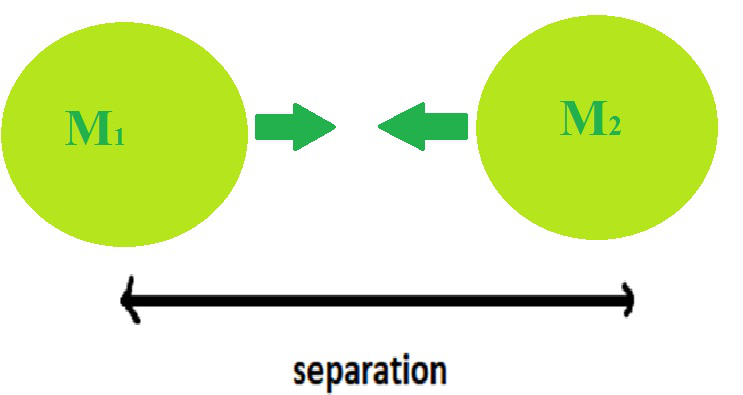### Sample Problems

Question 1. What is force?

A force can be defined as a push or pull upon an object resulting from the object’s interaction with another object. Whenever there is an interaction between two objects, there is a force upon each of the objects either in the same or opposite direction.

Question 2. What are 5 examples of force?

There are many examples of forces in our everyday lives:

• The force we exert upon hitting the ball with the bat,
• Force of the hair brush on hair when it is being brushed.
• Force of our foot pushing on the pedal when we ride a bicycle.
• Measuring the weight of any material.
• Using our muscular force to lift the bucket.

Question 3: What is the frictional force on an object if the coefficient of friction is 0.40 and the normal reaction offered is 50N.

As we know,Given, N= 50N0.40Hence the frictional force is 20N.

Question 4. What is the gravitational force on two bodies of masses 4kg and 2kg respectively which are at a distance of 4m and the universal gravitational constant being?

As we know,Putting the values from the question we get,On solving we getQuestion 5. What is the electrostatic force between two charges of charge 3C and 4C which are at a distance of 4m with a constant of proportionality 1?

As we know,Putting the given values from the question in the equation we getOn solving we get F=0.75N.

Question 6. What is the expression to calculate magnetic force?

Magnetic forces can be calculated by the following equationWhere, F= magnetic force

q= charge of moving particle

B= magnetic fieldangle between velocity and magnetic field vectors

My Personal Notes arrow_drop_up